HOME COURSES PREVIEW REVIEW ABOUT CONTACTToll-Free Info & Ordering M-F: 9am-5pm (PST): (877) RAPID-1024/7 Technical SupportQUICK TOURMember Login:Rapid Courses Catalog Mathematics in 24 Hours Chemistry in 24 Hours Biology in 24 Hours Physics in 24 HoursMath Survival Weekly
Get the insider's tips and tricks in how to survive your math course and ace the next test. Subscribe the Web's only math weekly newsletter for students and learn:
- How to Study Math Effectively
- How to Take Math Courses Strategically
- How to Solve Math Problems Systematically
- How to Score High in Math Exams
- How to Master Math Rapidly

Enter your name and email below and get started today!Math Study Lounge These study sheets are for quick review on the subjects. Refer to our rapid courses for comprehensive review.     - Getting Started with Algebra     - Geometry Basics     - How to Solve Math Problems     - Trigonometry Quick Review     - Statistics At-A-Glance     - Calculus PreviewHome » Trigonometry

Geometry Review

 Topic Review on "Title": Angles: An angle is either the figure formed by two rays starting from a common point or the measure of such a figure. Complimentary angles are two angles whose sum is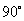. Supplementary angles are two angles whose sum is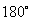. Congruent angles are angles with the same angle measurement. Vertical angles are angles at an intersection of two lines that are not adjacent to each other. Interior angles are two angles that are in the interior of a polygon. Exterior angles are two angles formed by the side of a polygon and an adjacent extends side or two angles on the outside of two parallel lines. Pythagorean Theorem: The Pythagorean Theorem is the sum of the squares for the legs of a right triangle equals the square of the hypotenuse, or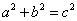. 30-60-90 Right Triangles: The sides of 30-60-90 right triangles are in the ratios of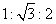. 45-45-90 Right Triangles: The sides of 45-45-90 right triangles are in the ratios of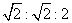. Regular polygon: A regular polygon is a geometric figure with three or more sides in which all interior angles are congruent. Area: Area is the size of a planar region or surface of a solid. Volume: Volume is the amount of space taken up by or enclosed by an object.

Rapid Study Kit for "Title":
 Flash Movie Flash Game Flash Card Core Concept Tutorial Problem Solving Drill Review Cheat Sheet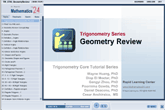"Title" Tutorial Summary : A review of geometric principles is covered in this tutorial. Most geometric relations can be fully understood with the use of the geometric figures and their properties. The formulation and development of area and volume concepts of geometric figures such as triangles and circles is covered in this tutorial. Similarity principles are introduced with the help of special triangles such as the 45-45-90 triangles.

 Tutorial Features: Specific Tutorial Features: • Step by step mathematical examples showing all details: Pythagorean theorem, 30-60-90 right triangles, 45-45-90 triangles, sum of interior angles, area of complex shapes Series Features: • Concept map showing inter-connections of new concepts in this tutorial and those previously introduced. • Definition slides introduce terms as they are needed. • Visual representation of concepts • Animated examples—worked out step by step • A concise summary is given at the conclusion of the tutorial.

 "Title" Topic List: ```Angles Angles and their definition Relationship of angles and their use Vertical angles Interior and exterior angles Geometric Shapes and Figures Right triangles Pythagorean Theorem 30-60-90 right triangles 45-45-60 right triangles 45-45-90 right triangles Regular polygons Area and Volume Definition of area and volume Area Formulas Volume Formulas ```

See all 24 lessons in Trigonometry, including concept tutorials, problem drills and cheat sheets:
Teach Yourself Trigonometry Visually in 24 Hours

 ©2014 Rapid Learning Center. Privacy Policy | Disclaimer Chemistry Survival Publishing, Biology Survival Publishing and Physics Survival Publishing are the divisions of Rapid Learning Inc.